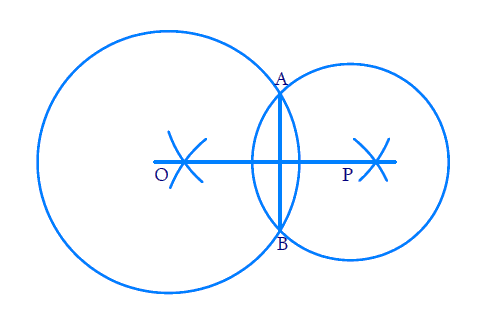# Ex.10.3 Q3 Circles Solution - NCERT Maths Class 9

Go back to  'Ex.10.3'

## Question

If two circles intersect at two points, prove that their centers lie on the perpendicular bisector of the common chord.

Video Solution
Circles
Ex 10.3 | Question 3

## Text Solution

What is known?

Two circles intersecting at two points.

What is unknown?

To check whether their centers lie on the perpendicular bisector of the common chord.

Reasoning:

By theorem $$10.4$$, it is known that perpendicular bisector of a chord passes through its centre. Since the chord is common to both the circles, the centre should lie on the perpendicular bisector of the chord.

Steps:Let the two circles intersect at point $${A}$$ and $${B}$$. Join $${AB}$$. $${AB}$$ is the common chord. By theorem $${10.4,}$$ it is known that perpendicular bisector of a chord passes through its centre.

As the chord $$AB$$ is common to both circles, the perpendicular bisector of the common chord should pass through their centers. So centre lies on the perpendicular bisector of the common chords.

Learn from the best math teachers and top your exams

• Live one on one classroom and doubt clearing
• Practice worksheets in and after class for conceptual clarity
• Personalized curriculum to keep up with school